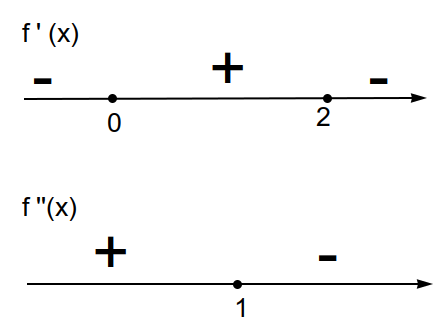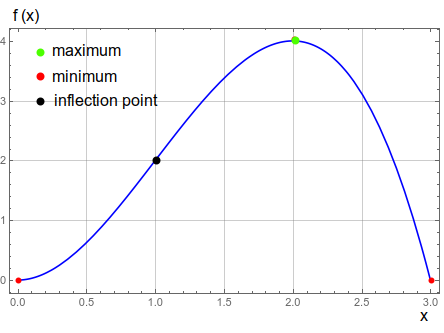# BIOMEDICAL: Dose-Response Curves. The relationship between the dosage, x, of a drug and the...

## Question:

BIOMEDICAL: Dose-Response Curves. The relationship between the dosage, {eq}x{/eq}, of a drug and the resulting change in body temperature is given by {eq}f(x) = x^2(3-x){/eq} for {eq}0 \leq x \leq 3{/eq}. Make sign diagrams for the first and second derivatives and sketch this dose-response curve, showing all relative extreme points and inflection points.

## Sign Diagrams

The sign diagrams are graphs where the relevant information is the sign of the function understudy, for each interval analyzed. If we have a function of one variable {eq}f(x) {/eq}, then the sign diagram is the numeric axis and over it, each interval has a sign associated with it. This diagram can be used to study inequalities.

We have the response function:

{eq}\begin{equation} f(x)=x^2(3-x) \end{equation} {/eq}

defined in the interval {eq}0\le x\le 3 {/eq}.

The first and second derivatives are:

{eq}\begin{align} f'(x)&=6 x - 3 x^2\\ f''(x)&=6-6x \end{align} {/eq}

The first derivative is positive when:

{eq}\begin{align} x(6-3x)>0\\ x(3x-6)<0\\ \end{align} {/eq}

Solving the equality we have:

{eq}\begin{align} x=0\\ x=2 \end{align} {/eq}

then the sign diagram (Figure 1) will be:and the first derivative is bigger than zero in the interval {eq}0<x<2 {/eq}. The points {eq}x=0,x=2 {/eq} are possibles extremes, and using the second derivative test we obtain that {eq}f''(0)>0,f''(2)<0 {/eq}. Therefore {eq}x=0 {/eq} is a minimum, while the point {eq}x=2 {/eq} is a maximum.

For the second derivative we have:

{eq}\begin{align} 6-6x>0\Rightarrow\;\;x<1 \end{align} {/eq}

The sign diagram is presented in Figure 1:

The point {eq}x=1 {/eq} is an inflection point, since the second derivative change sign around this point.

Also, the point {eq}x=3 {/eq} is an extreme of the function (frontier of the domain).

The sketch of the function is presented in Figure 2